Related Articles

# class std::string_view in C++17

• Last Updated : 09 Jun, 2021

The std::string has some demerits, one of the most common situations is constant strings. Below is the program that demonstrates the problem that occurs in dealing with constant strings with std::string:

Program 1:

## C++

 `// C++ program to demonstrate the``// problem occurred in string``#include ``#include ``using` `namespace` `std;` `// Driver Code``int` `main()``{``    ``char` `str_1[]{ ``"Hello !!, GeeksforGeeks"` `};` `    ``string str_2{ str_1 };``    ``string str_3{ str_2 };` `    ``// Print the string``    ``cout << str_1 << ``'\n'``         ``<< str_2 << ``'\n'``         ``<< str_3 << ``'\n'``;` `    ``return` `0;``}`

Output: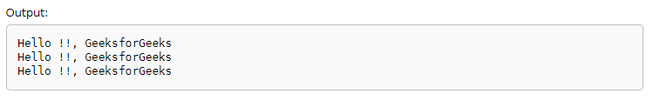Explanation: The output is the same as expected. But, in order to view “Hello !!, GeeksforGeeks” twice the std::string performs overheads on the memory twice. But here the task was to read the string (“Hello !!, GeeksforGeeks”), and no write operation is required on it. So just to display a string why assign memory multiple times. In order to deal with the strings more efficiently, C++17 proposed std::string_view() which provides the view of pre-defined char str[] without creating a new object to the memory.

Drawback of std::string: In the above example, the same string str_1 is printed twice by assigning two different string variables. So for both the variables str_2 and str_3, the memory was allocated using the static memory allocation, and that cause overhead on our memory twice.

Benefits of std::string_view:

• Light and Cheaper: The std::string_view is a very light, cheaper and is mainly used to provide the view of the string. Whenever the string_view is created there is no need to copy the string in the manner as done in the above example that was inefficient and was causing overhead on the memory. It makes the copying process of the string quite efficient and never creates any copy of the string when the modification is being made in the viewed string the made changes have appeared in the std::string_view.
• Better Performance: The std::string_view is better than the const std::string& because it removes the constraint of having a std::string object at the very beginning of the string as std::string_view is composed of two elements first one is const char* that points to the starting position of the array and the second is size.
• Supports crucial function: The std::string_view supports mostly all crucial function that is applied over the std::string like substr, compare, find, overloaded comparison operators (e.g., ==, <, >, !=). So in most of the cases, it removes the constraint of having a std::string object declaration when our preference is read-only.

std::string_view: C++17 library has proposed a standard type of string (std::string_view) which is different from the usual std::string.

• The std::string_view provides a lightweight object that offers read-only access to a string or a part of a string using an interface similar to the interface of std::_string and merely refers to the contiguous char sequence. Unlike std::string, which keeps its own copy of the string, It also provides a view of a string that is defined elsewhere in the source code.
• it is composed of two members: a const char* that points to the start of the char array, and the _size. It is a non-owning reference to a string in itself.
• It is defined in the header(#include <string_view>), and std::string_view class template is as follows:

template<class CharT, class Traits = std::char_traits<CharT>> class basic_string_view;

The class template string_view explains about an object that can refer to a constant contiguous sequence of char’s  or array of char’s -like objects with the first element of the sequence at position zero.

Below is the exact version of the above source code using std::string_view:

Program 2:

## C++

 `// C++ program to implement``// the above approach``#include ``using` `namespace` `std;``#include ` `// Driver code``int` `main()``{``    ``// View the text "hello", which is``    ``// stored in the binary``    ``string_view str_1{ ``"Hello !!, GeeksforGeeks"` `};` `    ``// View of the same "hello"``    ``string_view str_2{ str_1 };` `    ``// View of the same "hello"``    ``string_view str_3{ str_2 };` `    ``std::cout << str_1 << ``'\n '` `<< str_1 << ``'\n '` `<< str_3 << ``'\n'``;``    ``return` `0;``}`

Output-Explanation: The output would be the same as above, but no more copies of the string “Hello !!, GeeksforGeeks” are created in the Memory.

Parameters In std::string_view:

Char Type:
The char type defines the type of characters that are stored in the string_view. The C++ Standard Library provides the following typedefs for specializations of the template described above.

1. string_view for elements of type char
2. wstring_view, for wchar_t
3. u16string_view for char16_t
4. u32string_view for char32_t

Below is the C++ program to illustrate the Char Type:

Program 3:

## C++

 `// C++ program for the above approach``#include ``#include ``#include ``using` `namespace` `std;` `string_view sur_name(wstring_view x)``{``    ``return` `x.substr(6);``}` `// Driver Code``int` `main()``{``    ``// Basic_string_view wstr``    ``// (L"Madhav_Mohan"); both are equivalent``    ``const` `wchar_t``* str1{ L``"Madhav_Mohan"` `};` `    ``cout << your_name(str1);` `    ``return` `0;``}`

Output-Example Of std::string_view: Below examples, show that most of the string-based functions can be used with std::string_view as used str.compare(), str.back(), str.cend(), and str.at() functions.

Program 4:

## C++

 `// C++ program to implement``// the above approach``#include ``#include ``using` `namespace` `std;` `string to_alpha(``int` `r)``{``    ``if` `(r < 0)``        ``return` `" less than "``;``    ``else` `if` `(r == 0)``        ``return` `" equal to "``;``    ``else``        ``return` `" greater than "``;``}` `// Driver code``int` `main()``{``    ``char` `str{ ``"Muddy"` `};``    ``char` `str_1{ ``"Maddy"` `};` `    ``string_view str_2{ str_1 };` `    ``// This line prints Maddy``    ``cout << str_2 << ``'\n'``;` `    ``// Change 'M' to 'D' in arr``    ``str_1 = ``'D'``;` `    ``// This line prints Daddy``    ``cout << str_2 << ``'\n'``;` `    ``cout << str_2.at(2);``    ``cout << str_2.back();``    ``cout << ``" is last char of str_2 is y "` `<< (str_2.back() == ``'s'``);``    ``cout << ``" is last char of str_2 is y "` `<< boolalpha << (str_2.back() == ``'s'``);` `    ``// In above statement boolalpha is``    ``// used to  give the boolean result``    ``// in True or False` `    ``int` `cmp{ str_1.compare(str_2) };` `  ``cout << to_alpha(cmp``};``int` `cmp_1 = str.compare(1, 4, str_2);``cout << ``"last three characters of str are"` `<< to_alpha(cmp_1) << ``"str_2.\n"``;``return` `0;``}`

Output: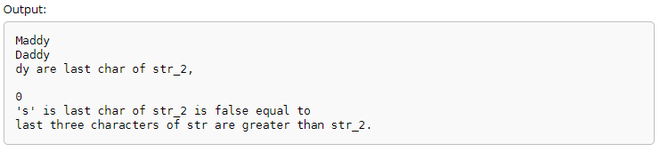Modification of std::string_view: In C++20 some new functions have been added like str.remove_suffix(), str.remove_suffix() using these functions can modify the std::string_view. Like can remove the selective suffix or prefixes of the given string.

Program 5:

## C++

 `// C++ program to implement``// the above approach``#include ``#include ``#include ``using` `namespace` `std;` `// Driver code``int` `main()``{``    ``string_view str_1{ ``"Maddy"` `};``    ``cout << str_1 << ``'\n'``;` `    ``string_view str_2{ ``"Daddy"` `};``    ``cout << str_2 << ``'\n'``;` `    ``string_view var{ ``"Hello !!, GeeksforGeeks"` `};``    ``cout << var << ``'\n'``;` `    ``cout << var.starts_with(``"Hi !!"``) << ``'\n'``;``    ``cout << var.ends_with(``"GeeksforGeeks !!"``) << ``'\n'``;` `    ``// Remove the first characters.``    ``str_1.remove_prefix(1);``    ``cout << str_1 << ``'\n'``;` `    ``// Remove the last 2 characters.``    ``str_1.remove_suffix(2);``    ``std::cout << str_1 << ``'\n'``;` `    ``return` `0;``}`

Output: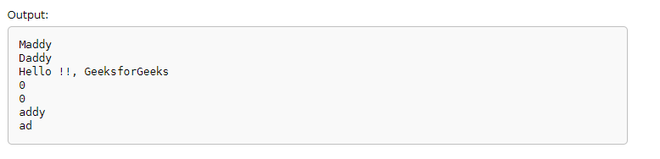Below is the example of some other operations that can be performed with std::string_view:

Program 6:

## C++

 `// C++ program to implement the``// above approach``#include ``#include ``#include ``#include ``#include ``#include ``using` `namespace` `std;` `// Driver code``int` `main()``{``    ``constexpr string_view str{ ``"Daddy"` `};``    ``constexpr string_view str1{ ``"Maddy"` `};``    ``string str2{};` `    ``// Copy data of str1 into str2``    ``str1.copy(str2.data(), 4);` `    ``// This line prints Madddy``    ``cout << str2.data() << ``'\n'``;` `    ``if` `((str.compare(str2)) != 0)``        ``cout << str << ``" "``             ``<< ``"doesn't = to"``             ``<< ``" "` `<< str1 << ``'\n'``;``    ``cout << str1.starts_with(``"Mad"``);``    ``cout << ``'\n'``         ``<< str.starts_with(``"Pad"``) << ``'\n'``;``    ``cout << str1.ends_with(``"ddt"``);``    ``cout << ``'\n'``         ``<< str.ends_with(``"ddy"``) << ``'\n'``;` `    ``// Checks whether the given string contains``    ``// the given substring or character``    ``constexpr string_view s1{ ``"Hello! Madhav"` `};` `    ``// Position of the first character that``    ``// match first``    ``constexpr string_view str3{ ``"Hi! Maddy"` `};` `    ``size_t` `found = str3.find(str1);``    ``cout << found << ``'\n'``;` `    ``// Find the last occurrence of a substring``    ``cout << ``'\n'``         ``<< str3.rfind(``'d'``);` `    ``// Gives the first time occured char's position``    ``cout << ``'\n'``         ``<< str3.find_first_of(``"d"``);` `    ``// Gives the last time occured char's position``    ``cout << ``'\n'``         ``<< str3.find_last_of(``"d"``);` `    ``// Finds the first char that's not equal to``    ``// any of the char(s) in the given string``    ``cout << ``'\n'``         ``<< str3.find_last_not_of(str, 6);` `    ``// It givesIndex of first unmatched character``    ``// when successful or string::npos if no such``    ``// character found. It searches for the first``    ``// char that doesn't match any of the char(s)``    ``// that has given in the argument``    ``cout << ``'\n'``         ``<< str3.find_last_not_of(str, 4);` `    ``return` `0;``}`

Output: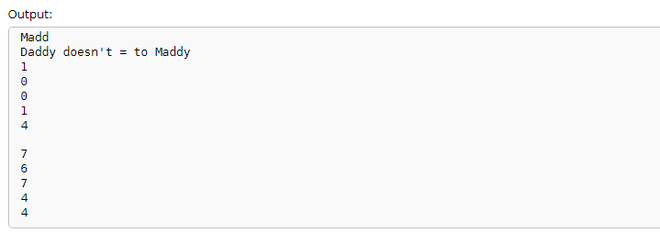Non-null Terminated Strings: Unlike C-strings and std::string which need a string terminator (‘\0’) at the end of a string, std::string_view doesn’t need null terminators to mark the end of the string. Because it keeps the track of the string length.

Below is the C++ program to implement the above approach:

Program 7:

## C++

 `// C++ program to implement``// the above approach``#include ` `// For std::size``#include ``#include ``using` `namespace` `std;` `// Driver code``int` `main()``{``    ``// No null-terminator.``    ``char` `name[]{ ``'M'``, ``'a'``, ``'d'``,``                 ``'h'``, ``'a'``, ``'v'` `};` `    ``// Here name isn't null-terminated.``    ``// We need to pass the length manually.``    ``// Because name is an array, we can``    ``// use std::size to get its length.``    ``string_view str_1{ \name, size(name) };` `    ``// This is safe and cout knows how to``    ``// print std::string_views.``    ``cout << str_1 << ``'\n'``;` `    ``return` `0;``}`

Output:Conversion Of std::string_view Into std::string: Explicit conversion methods need to be used because implicit conversion won’t help here.

Below is the C++ program to implement the conversion of std::string_view to std::string:

Program 8:

## C++

 `// C++ program to implement``// the above approach``#include ``#include ``#include ``using` `namespace` `std;` `void` `print(string s)``{``    ``cout << s << ``'\n'``;``}` `// Driver code``int` `main()``{``    ``string_view str_1{ ``"Madhav"` `};``    ``str_1.remove_suffix(3);` `    ``// compile error: won't implicitly``    ``// convert``    ``// print(str_1);` `    ``// explicit conversion``    ``string str_2{ str_1 };` `    ``print(str_2);` `    ``// It'll work``    ``print(``static_cast``(str_1));` `    ``return` `0;``}`

Output: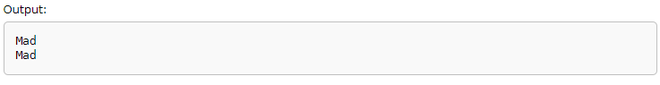Conversion Of std::string_view To C-style String: Functions such as strlen(), these functions need a C-style string in order to be used. So, whenever there is a need to convert a std::string_view to a C-style string, it can be done by first converting to a std::string.

Below is the C++ program to implement the above approach:

Program 9:

## C++

 `// C++ program to implement``// the above approach``#include ``#include ``#include ``#include ` `// Driver code``int` `main()``{``    ``string_view str_1{ ``"Madhav"` `};``    ``str_1.remove_suffix(3);` `    ``// Create a std::string from``    ``// the std::string_view``    ``string str_2{ str_1 };` `    ``// A Null_terminated C-style string.``    ``auto` `Null_Terminated{ str_2.c_str() };` `    ``// Pass the null-terminated string``    ``// to the function that we want to use.``    ``cout << str_2 << ``" has "` `<< ``strlen``(Null_Terminated) << ``" letter's\n"``;` `    ``return` `0;``}`

Output: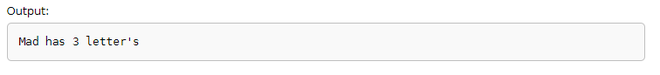Scoping Issues With std::string_view: The std::string_view provides a view, so it remains independent of a string of which it provides a view, and it projects the view of the string unless the viewed string is in scope but in case if the viewed strings scope goes out then the std::string_view has nothing that remains to project so it shows undefined behavior. std::string_view always needs a string literal or a string that has been created by a std::string within the scope in order to project the view of string, but as soon as scope dies std::string_view cannot create any strings on its own because it’s just only a view.

Below is the example to implement the above approach:

Program 10:

## C++

 `// C++ program to implement``// the above approach``#include ``#include ``#include ``using` `namespace` `std;` `string_view ask_name()``{``    ``cout << ``"Write your surname?\n"``;` `    ``// A std::string is needed, because``    ``// std::cin needs to modify it.``    ``string str_1{};``    ``cin >> str_1;` `    ``// Pass str_1 to std::string_view``    ``string_view str_2{ str_1 };` `    ``cout << str_2 << ``'\n'``;` `    ``// str_1 dies, and so does the``    ``// string that str created.``    ``return` `str_2;``}` `// Driver code``int` `main()``{``    ``std::string_view str_2{ ask_name() };` `    ``// str_2 is observing a string that``    ``// already died.` `    ``// Undefined behavior you'll observe``    ``cout << your surname is << str_2 << ``'\n'``;` `    ``return` `0;``}`

Output: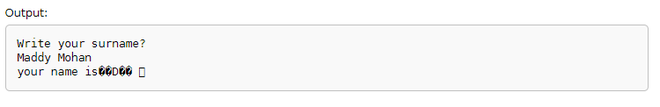Execution Of std::string_view with data() Function: The data() function writes the characters of the string into an array. It returns a pointer to the array, obtained from the conversion of string to the array. Its Return type is not a valid C-string as no ‘\0’ character gets appended at the end of the array.

Below is the C++ program to implement the above approach:

Program 11:

## C++

 `// C++ program to implement``// the above approach``#include ``#include ``#include ``using` `namespace` `std;``#include ` `// Driver code``int` `main()``{``    ``string str{ ``"Madhav_Mohan"` `};``    ``string_view str1{ str.data() };``    ``cout << str1 << endl;``    ``string_view str2{ str1.substr(6) };``    ``string_view str3{ str2 };``    ``cout << str3;``    ``return` `0;``}`

Output: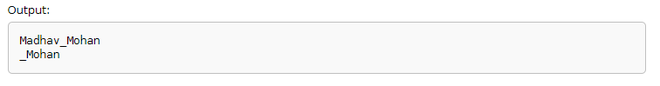Non-member functions:

```   operator==
operator!=                                    (has been removed in C++20)
operator<                                     (has been removed in C++20)
operator>                                     (has been removed in C++20)
operator<=                                    (has been removed in C++20)
operator>=                                    (has been removed in C++20)
operator<=>                                   (has newly been included C++20)```

Note: Std::string_view run only in C++17 or in above versions only. There is a number of functions that are incorporated with std::string_view some of them are new(C++20) and some of them are old. Here below I am referencing the web_address where you can get them too into deep.

Want to learn from the best curated videos and practice problems, check out the C++ Foundation Course for Basic to Advanced C++ and C++ STL Course for foundation plus STL.  To complete your preparation from learning a language to DS Algo and many more,  please refer Complete Interview Preparation Course.

My Personal Notes arrow_drop_up